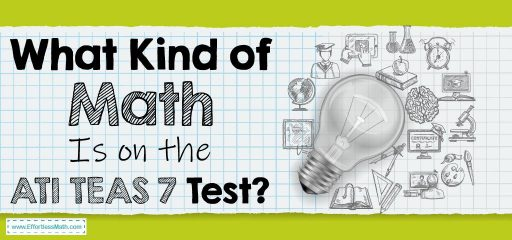# What Kind of Math Is on the ATI TEAS 7 Test?The ATI TEAS 7 (Test of Essential Academic Skills) has been published in 2016 by the Assessment Technologies Institute (ATI) to assess students’ ability to enter health sciences. This test, known as TEAS 7, consists of 4 sections as follows:

• Reading: 53 Questions – 64 Minutes
• Mathematics: 36 Questions – 54 Minutes
• Science: 53 Questions – 63 Minutes
• English and Language Usage: 28 Questions – 28 Minutes

This test has 170 questions, all of which are multiple-choice. The total time to answer the questions is 209 minutes. The mathematics section has two main sections: Number and Algebra, Measurement, and Data. For the math section, students are allowed to use a four-function calculator. In the following section, we take a brief look at the contents of each math section of the ATI TEAS 7  test.

## Numbers and Algebra

In Numbers and Algebra, you must first strengthen your algebra skills and knowledge. You may encounter questions in the form of statements, you should be able to turn them into equations and other forms of a mathematical expression. You must also be able to convert one type of ratio, such as percentage or fraction, to another. In this section, you will also come across questions related to word problems. Other topics covered in this section include rational numbers, proportions, percentages, estimation, and single variable equations. To succeed in this part of the math test, you must pay close attention to the wording of each problem. Carefully review the questions, you need to know what you are asked and how to begin the process of solving. In this case, you can get the best result in this section.

## Measurement and Data

In the Measurement and Data section, you will encounter relatively complex questions. You need to be able to analyze quantitative data in different ways. Reading graphical data, such as graphs and tables, and interpreting them is very important in this section. Solving geometric equations to find specific measurements is also very important. Knowing the geometric properties and which formulas work best in which questions, can help you a lot. In this section, you should be able to describe how a pair of variables interact with one another. To succeed in this section and get the desired score, be sure to review both metric and standard units of measurement. You should also review arithmetic and other steps related to switching from one system to another.

No Product Here.

## FAQs:

### Is the ATI TEAS 7 Math test hard?

This test can be as difficult as any other. However, with enough study and practice, you can succeed in this test.

### How do I study for the ATI TEAS 7 Math?

How you prepare for the ATI TEAS 7 Math test is very important. You can use good prep books to prepare well for the ATI TEAS 7 Math test. You can also use online practice tests for further practice after studying the ATI TEAS 7 exam courses.

### How is the ATI TEAS 7 Test Scored?

The ATI TEAS test uses a grading system in which test questions are given a variety of point values. This grading system is known as equivalence. for more information, you can check here.

## The Best Books to Ace the ATI TEAS 7 Math Test

### What people say about "What Kind of Math Is on the ATI TEAS 7 Test? - Effortless Math: We Help Students Learn to LOVE Mathematics"?

No one replied yet.

X
52% OFF

Limited time only!

Save Over 52%

SAVE \$40

It was \$76.99 now it is \$36.99